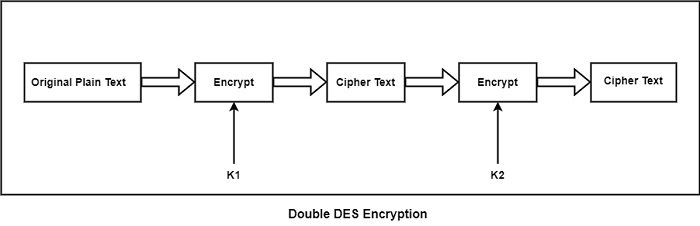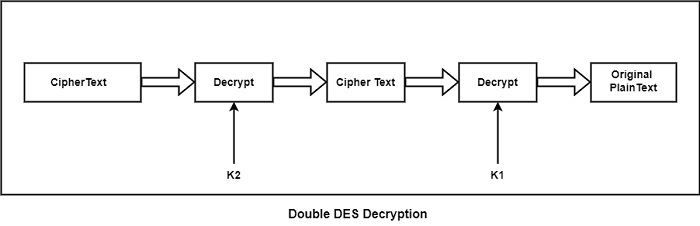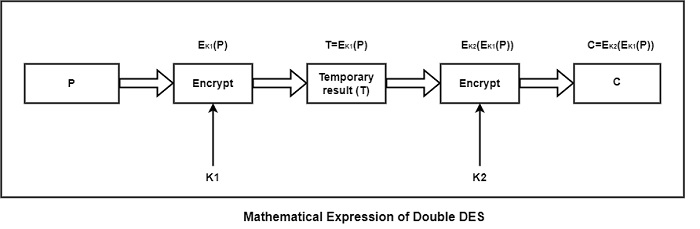# What is Double DES?

The Data Encryption Standard (DES) is a symmetric key block cipher which creates 64-bit plaintext and 56-bit key as an input and makes 64-bit cipher text as output. The DES function is create up of P and S-boxes. P-boxes transpose bits and S-boxes substitute bits to make a cipher.

DES is a Feistel Block Cipher implementation, called a LUCIFER. It need a Feistel structure with 16 rounds, where a different key can be used for each round. The major reasons to understand DES (Data Encryption Standard) is that it forms the foundation for encryption algorithms. This creates it easy for one to learn the implementation or operating of currently used encryption algorithms or methods, which are much quicker than the DES algorithm.

Double DES is an encryption approach which uses two example of DES on same plain text. In both examples it provides different keys to encode the plain text. Double DES is easily to learn.

Double DES uses two keys, such as k1and k2. It can implement DES on the original plain text using k1 to get the encrypted text. It can implement DES on the encrypted text, but this time with the different key k2. The final output is the encryption of encrypted text as shown in the figure.The double encrypted cipher-text block is first decrypted using the key K2 to make the singly encrypted cipher text. This ciphertext block is then decrypted using the key K1 to acquire the original plaintext block.If it can use a key of only 1 bit, there are two possible keys including 0 and 1. If it can use a 2 bit key, there are four possible key values such as (00, 01, 10 and 11).

In general, if it can use an n-bit key, the cryptanalyst has to implement 2n operations to try out all the possible keys. If it can use two different keys, each including n bits, the cryptanalyst would require 22n attempt to crack the key.

Double DES needed a key search of (22*56), i. e. , 2112keys. It introduce the terms of the meet-in-the-middle attack. This attack contains encryption from one end, decryption from the other and connecting the results in the middle.

Consider that the cryptanalyst understand two basic pieces of information including P (a plain-text block) and C (the corresponding final cipher-text block) for a message. The numerical expression of Double DES as shown in the figure.

The result of the first encryption is known as T and is indicated as T = Ek1(P) [i.e., encrypt the block P with key K1]. After this encrypted block is encrypted with another key K2, it indicate the result as C = EK2(EK1(P)) [i.e., encrypt the already encrypted block T, with a different key K2, and call the final ciphertext as C].